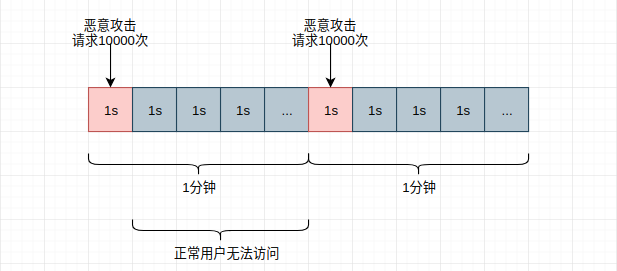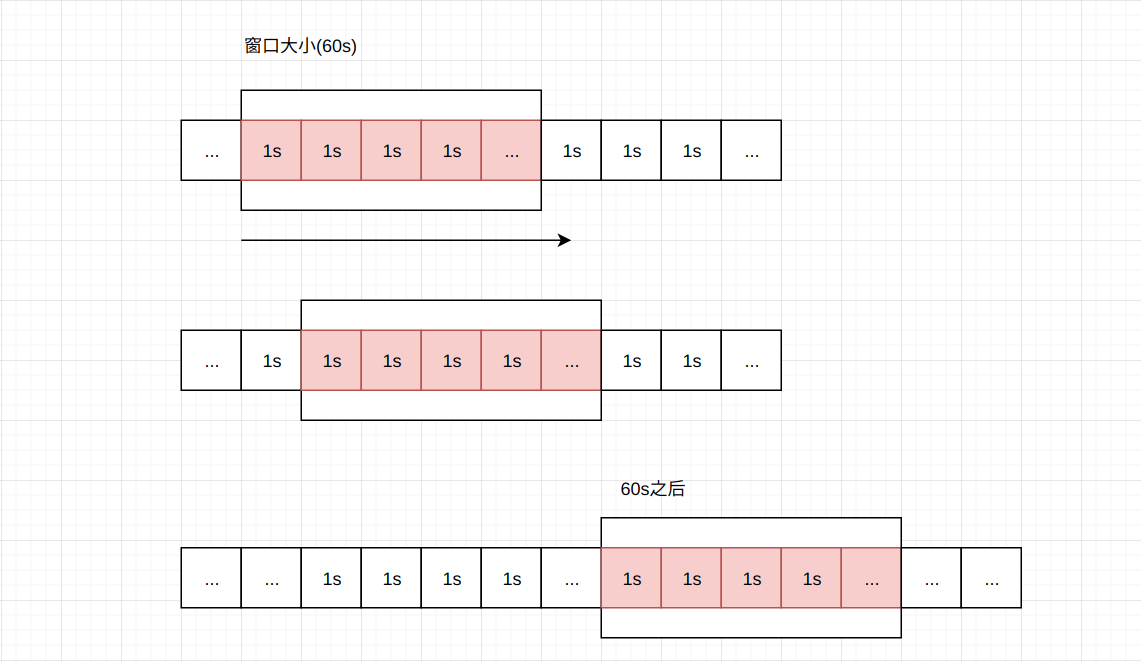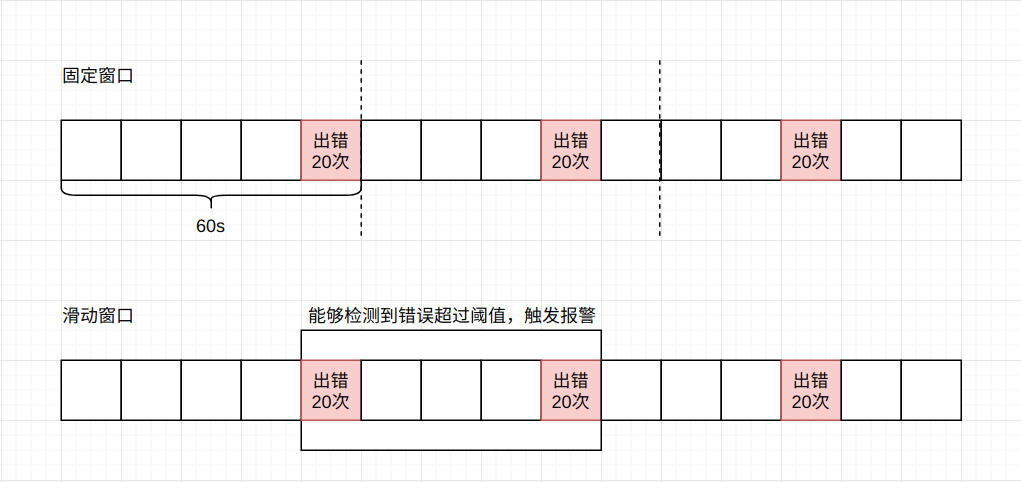#### 1. 固定窗口

``````# 该功能可用Lua优化，详见Lua模块
from redis import Redis
redis = Redis("127.0.0.1", 6379)

def hit_user_access(api):
try:
redis_key = "restrict_access:"+api
access_number = redis.incr(redis_key)
if access_number == 1:
redis.expire(redis_key, 60)
if access_number > MAX_ACCESS_NUMBER:
return True
return False
except Exception as e:
# 日记记录
print(e)
``````#### 2. 滑动窗口``````local key = KEYS

local now_timestamp = tonumber(ARGV)
local window_size = tonumber(ARGV)
local limit = tonumber(ARGV)

local should_clear = now_timestamp - window_size
redis.call("ZREMRANGEBYSCORE", key, 0, should_clear)

local amount = redis.call("ZCARD", key)
if amount < limit then
end
redis.call("EXPIRE", key, window_size)

return amount < limit
``````

``````class MovingWindow(object):

def __init__(self, window, rate):
"""
:param window: 窗口大小
:param rate: 移动速率
"""
assert window > 0 and rate > 0, "window and rate should more than zero value"
assert isinstance(window, int) and isinstance(rate, int), "window and rate should be a Integer"
self.window = window
self.rate = rate

self.__window =  * self.window
self.__last_moving = int(time.time())

def __shift(self, step):
"""
:param step: 窗口向右滑动的距离
"""
if step > self.window:
self.__clear()
self.__window = self.__window[step:] +  * step

def __clock_shift(self):
"""
计算窗口应当滑动的距离
"""
now = int(time.time())
expire = now - self.__last_moving
step = int(expire / self.rate)
if step > 0:
self.__shift(step)
self.__last_moving = now

def incr(self, value):
# 首先将窗口滑动至正确位置
self.__clock_shift()
# 将值添加至窗口最后一个元素上即可
self.__window[-1] += value

def count(self):
"""
:return: 返回当前窗口计数总数
"""
self.__clock_shift()
return sum(self.__window)

def __clear(self):
self.__window =  * self.window

def __repr__(self):
return "window: {}".format(self.__window)
``````

#### 3. 令牌桶

``````class TokenBucket(object):

def __init__(self, capacity, rate):
"""
:param capacity: 桶的容量
:param rate: 生成令牌的速率
"""
assert capacity > 0 and rate > 0, "capacity and rate should more than zero"
assert isinstance(capacity, int) and isinstance(rate, int), "capacity and rate should be integer"
self.capacity = capacity
self.rate = rate
self.__last_clock = int(time.time())
# 初始令牌数为0
self.__bucket = 0

def hit(self):
# 非并发安全的实现
now = int(time.time())
self.__bucket = min(self.capacity, (now - self.__last_clock) * self.rate)
if self.__bucket < 1:
return True
else:
self.__bucket -= 1
self.__last_clock = now
return False
``````